Displays a waterfall break down plot for objects of break_down class.

# S3 method for break_down
plot(
x,
...,
baseline = NA,
max_features = 10,
min_max = NA,
vcolors = DALEX::colors_breakdown_drwhy(),
digits = 3,
rounding_function = round,
shift_contributions = 0.05,
plot_distributions = FALSE,
vnames = NULL,
title = "Break Down profile",
subtitle = "",
max_vars = NULL
)

## Arguments

x an explanation created with break_down other parameters. if numeric then veritical line starts in baseline. maximal number of features to be included in the plot. default value is 10. a range of OX axis. By default NA, therefore it will be extracted from the contributions of x. But it can be set to some constants, useful if these plots are to be used for comparisons. If NA (default), DrWhy colors are used. number of decimal places (round) or significant digits (signif) to be used. See the rounding_function argument. a function to be used for rounding numbers. This should be signif which keeps a specified number of significant digits or round (which is default) to have the same precision for all components. if TRUE, variable contributions will be added to the plot number describing how much labels should be shifted to the right, as a fraction of range. By default equal to 0.05. if TRUE then distributions of conditional propotions will be plotted. This requires keep_distributions=TRUE in the break_down, local_attributions, or local_interactions. a character vector, if specified then will be used as labels on OY axis. By default NULL a character. Plot title. By default "Break Down profile". a character. Plot subtitle. By default "". alias for the max_features parameter.

## Value

a ggplot2 object.

Explanatory Model Analysis. Explore, Explain and Examine Predictive Models. https://ema.drwhy.ai

## Examples

library("DALEX")
library("iBreakDown")
set.seed(1313)
model_titanic_glm <- glm(survived ~ gender + age + fare,
data = titanic_imputed, family = "binomial")
explain_titanic_glm <- explain(model_titanic_glm,
data = titanic_imputed,
y = titanic_imputed$survived, label = "glm") #> Preparation of a new explainer is initiated #> -> model label : glm #> -> data : 2207 rows 8 cols #> -> target variable : 2207 values #> -> predict function : yhat.glm will be used ( default ) #> -> predicted values : No value for predict function target column. ( default ) #> -> model_info : package stats , ver. 4.1.1 , task classification ( default ) #> -> predicted values : numerical, min = 0.1490412 , mean = 0.3221568 , max = 0.9878987 #> -> residual function : difference between y and yhat ( default ) #> -> residuals : numerical, min = -0.8898433 , mean = 4.198546e-13 , max = 0.8448637 #> A new explainer has been created! bd_glm <- break_down(explain_titanic_glm, titanic_imputed[1, ]) bd_glm #> contribution #> glm: intercept 0.322 #> glm: gender = male -0.107 #> glm: fare = 7.11 -0.018 #> glm: age = 42 -0.014 #> glm: class = 3rd 0.000 #> glm: embarked = Southampton 0.000 #> glm: sibsp = 0 0.000 #> glm: parch = 0 0.000 #> glm: survived = 0 0.000 #> glm: prediction 0.183plot(bd_glm, max_features = 3)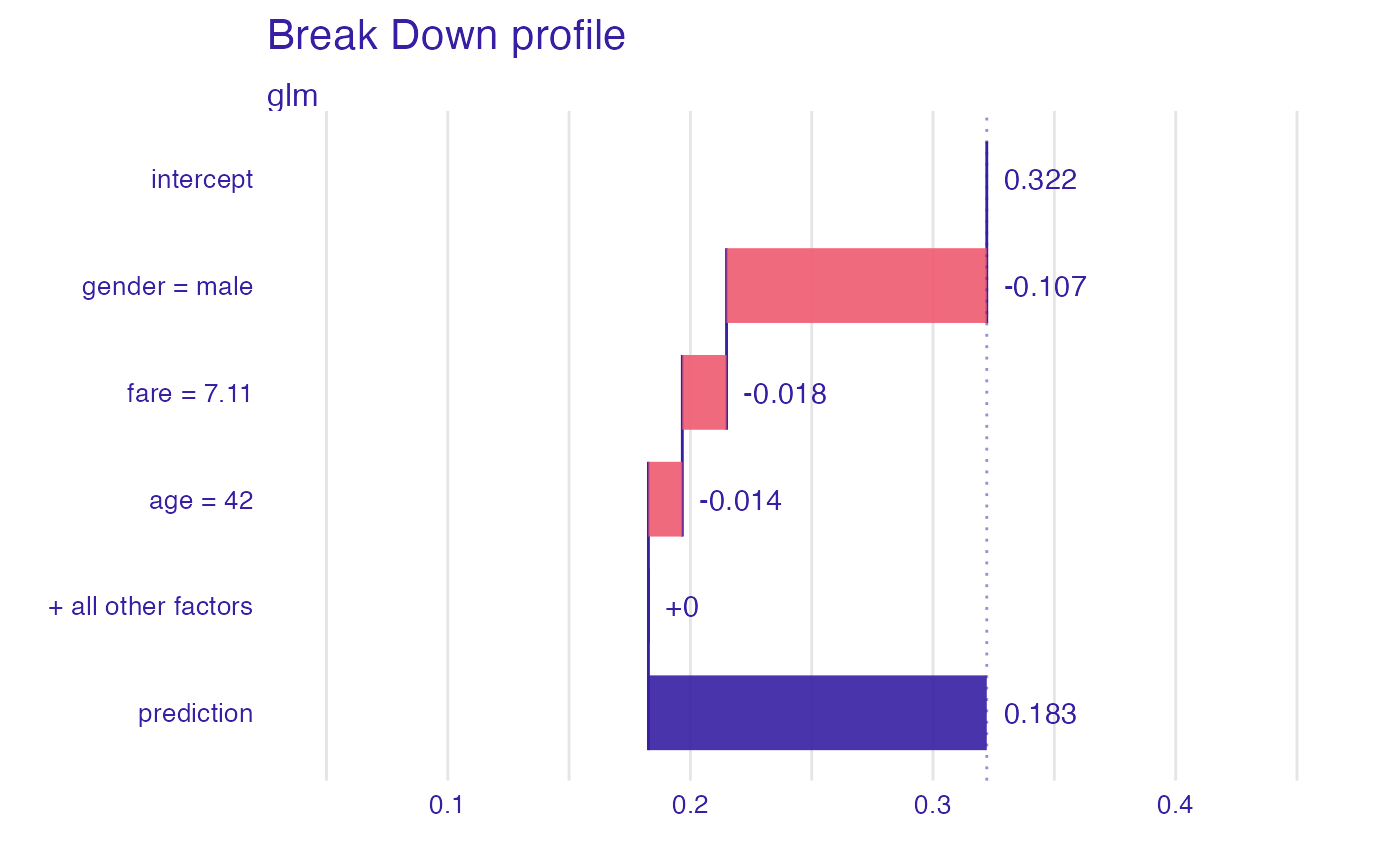plot(bd_glm, max_features = 3, vnames = c("average","+ male","+ young","+ cheap ticket", "+ other factors", "final"))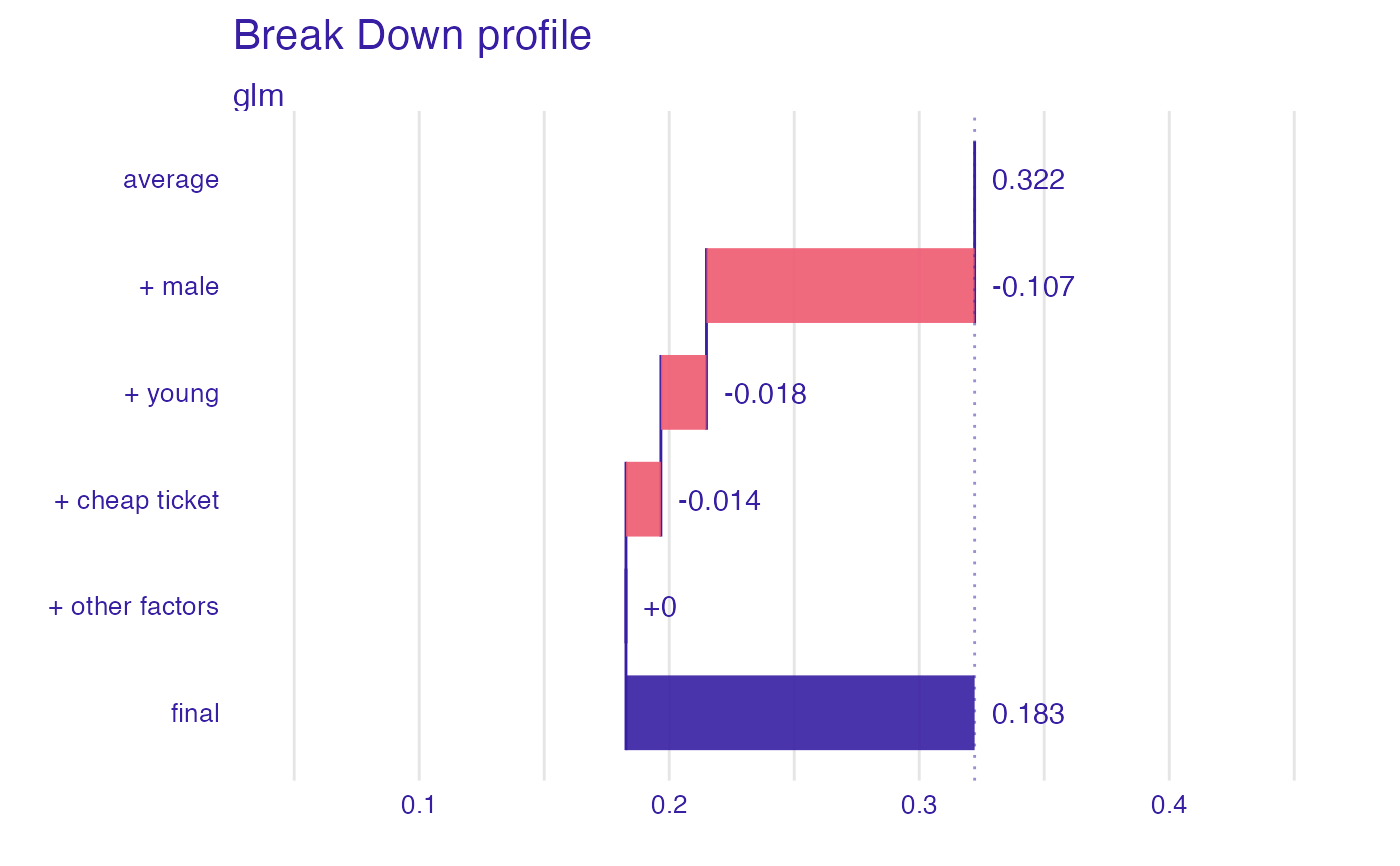# \dontrun{ ## Not run: library("randomForest") set.seed(1313) # example with interaction # classification for HR data model <- randomForest(status ~ . , data = HR) new_observation <- HR_test[1,] explainer_rf <- explain(model, data = HR[1:1000,1:5]) #> Preparation of a new explainer is initiated #> -> model label : randomForest ( default ) #> -> data : 1000 rows 5 cols #> -> target variable : not specified! ( WARNING ) #> -> predict function : yhat.randomForest will be used ( default ) #> -> predicted values : No value for predict function target column. ( default ) #> -> model_info : package randomForest , ver. 4.6.14 , task multiclass ( default ) #> -> model_info : Model info detected multiclass task but 'y' is a NULL . ( WARNING ) #> -> model_info : By deafult multiclass tasks supports only factor 'y' parameter. #> -> model_info : Consider changing to a factor vector with true class names. #> -> model_info : Otherwise I will not be able to calculate residuals or loss function. #> -> predicted values : predict function returns multiple columns: 3 ( default ) #> -> residual function : difference between 1 and probability of true class ( default ) #> A new explainer has been created! bd_rf <- local_attributions(explainer_rf, new_observation) bd_rf #> contribution #> randomForest.fired: intercept 0.386 #> randomForest.fired: hours = 42.32 0.231 #> randomForest.fired: evaluation = 2 0.062 #> randomForest.fired: salary = 2 -0.272 #> randomForest.fired: age = 57.73 0.092 #> randomForest.fired: gender = male 0.281 #> randomForest.fired: prediction 0.778 #> randomForest.ok: intercept 0.278 #> randomForest.ok: hours = 42.32 -0.053 #> randomForest.ok: evaluation = 2 0.091 #> randomForest.ok: salary = 2 0.271 #> randomForest.ok: age = 57.73 -0.086 #> randomForest.ok: gender = male -0.283 #> randomForest.ok: prediction 0.218 #> randomForest.promoted: intercept 0.336 #> randomForest.promoted: hours = 42.32 -0.178 #> randomForest.promoted: evaluation = 2 -0.152 #> randomForest.promoted: salary = 2 0.001 #> randomForest.promoted: age = 57.73 -0.006 #> randomForest.promoted: gender = male 0.002 #> randomForest.promoted: prediction 0.004plot(bd_rf)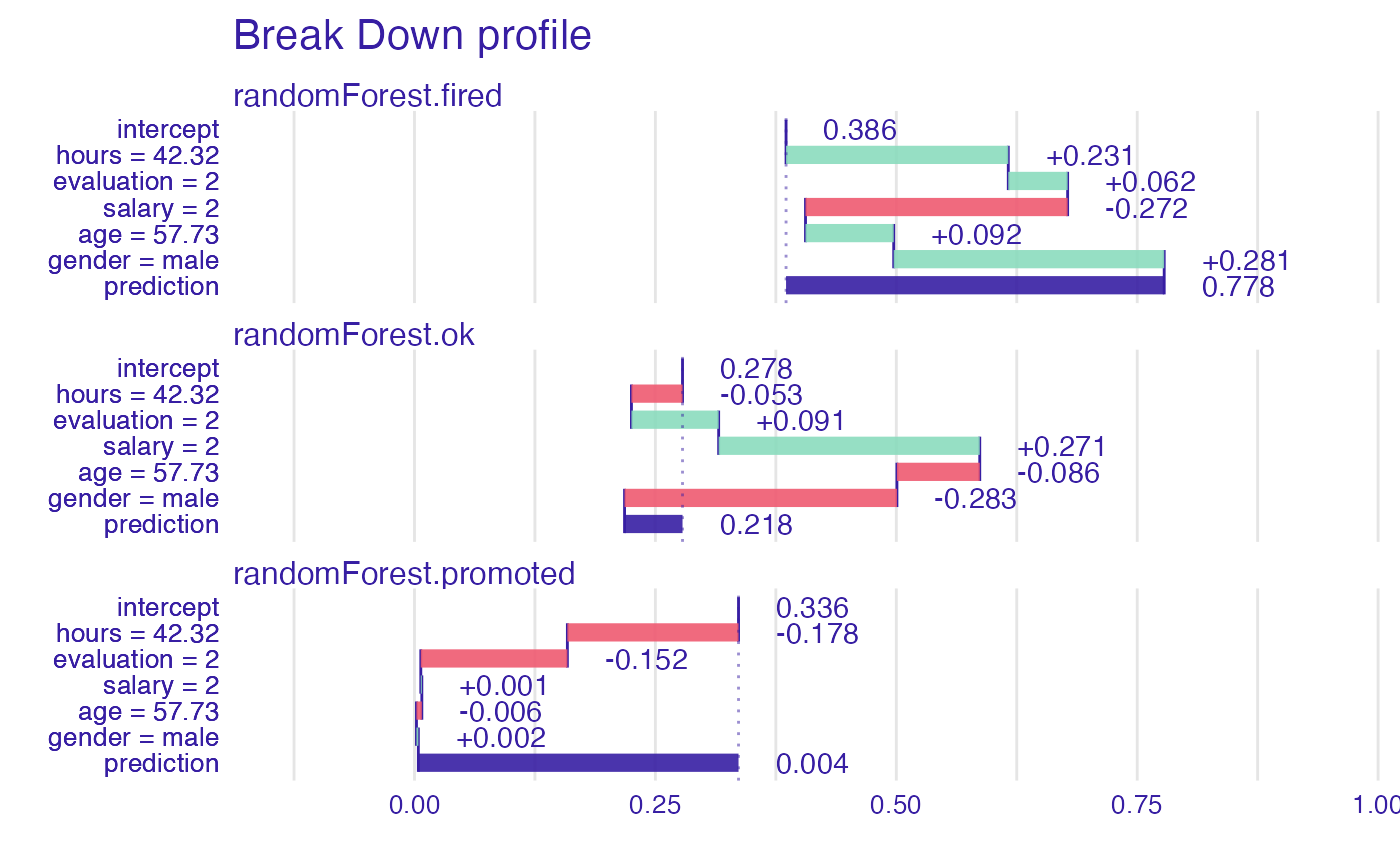plot(bd_rf, baseline = 0)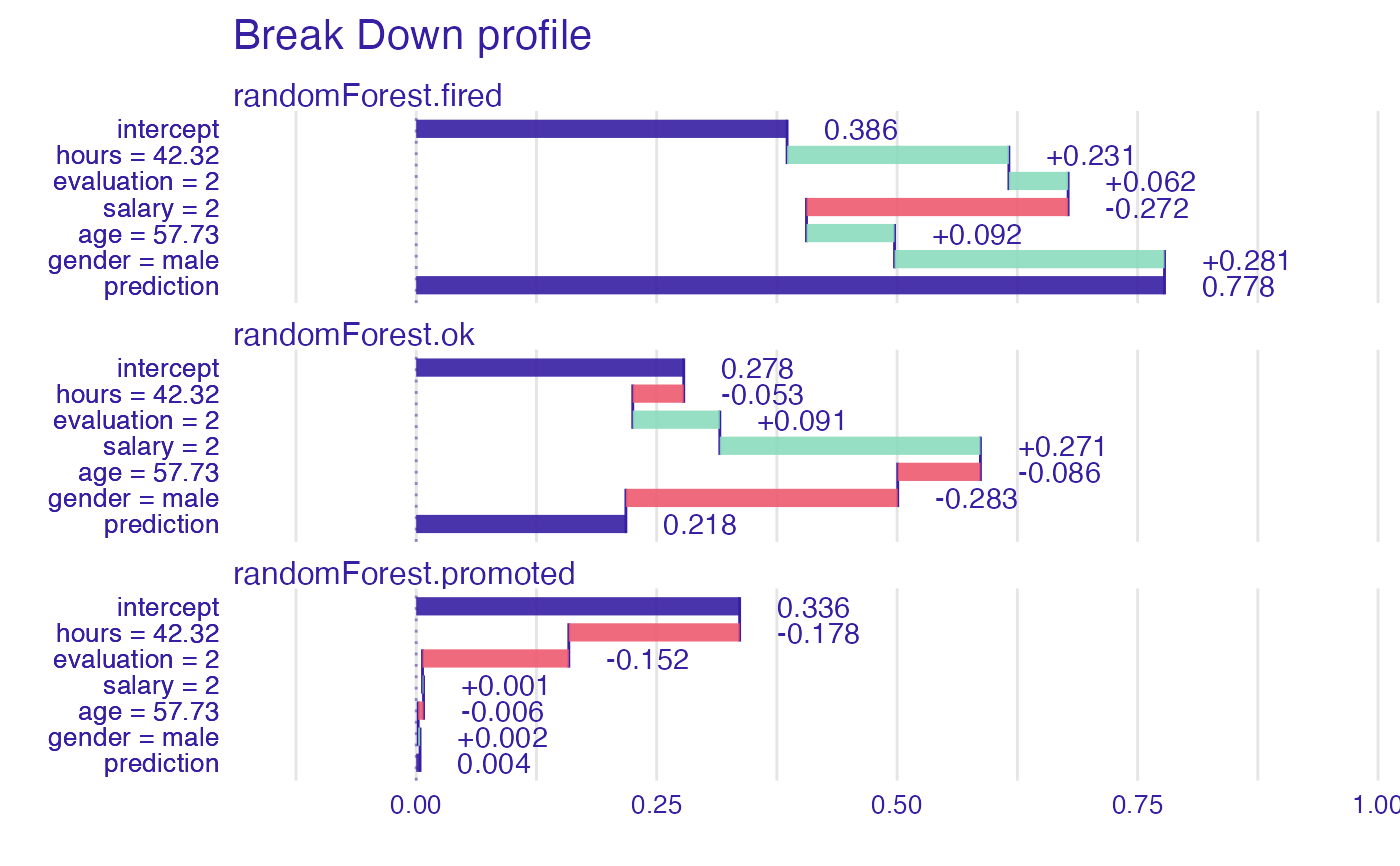plot(bd_rf, min_max = c(0,1))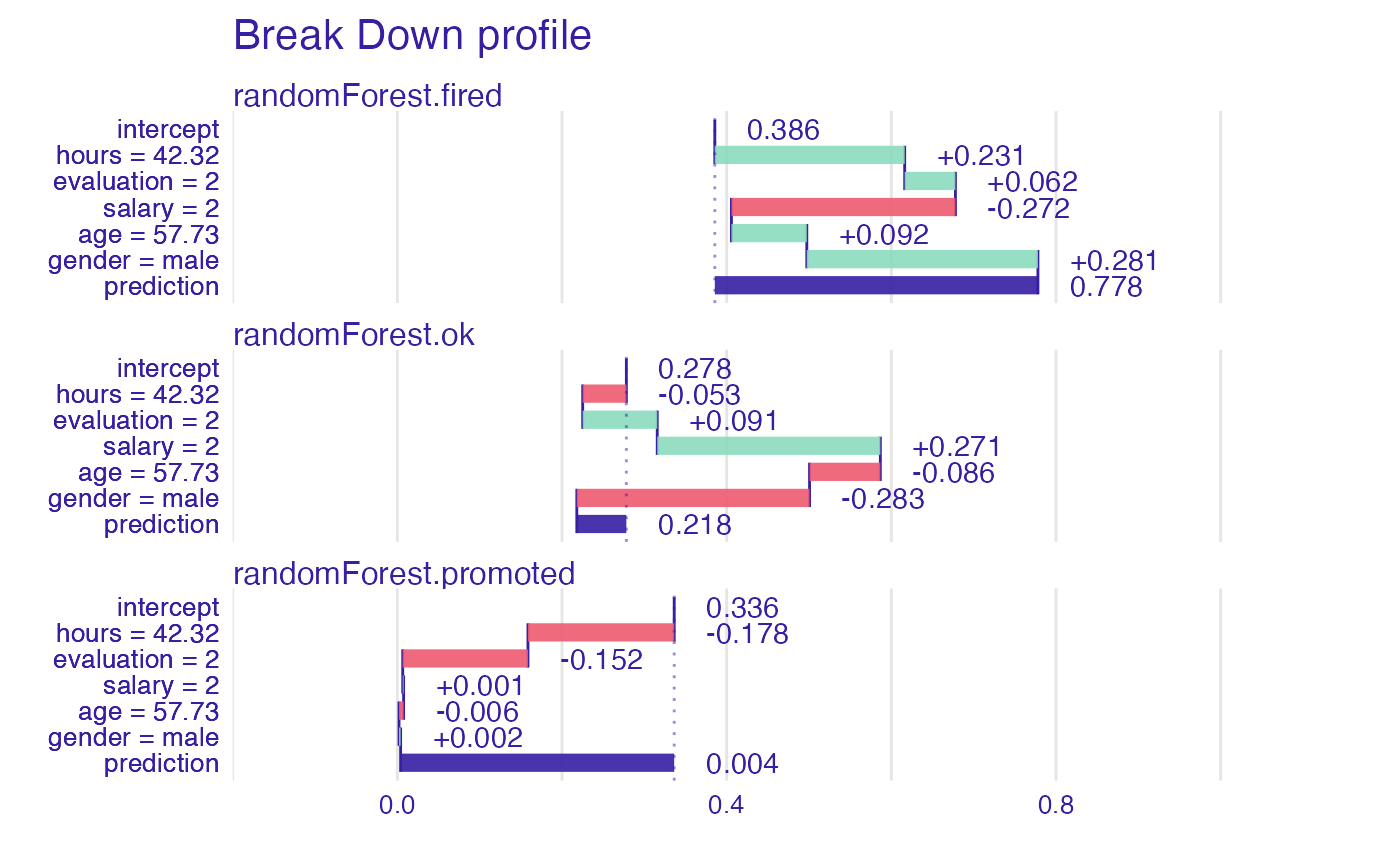bd_rf <- local_attributions(explainer_rf, new_observation, keep_distributions = TRUE) bd_rf #> contribution #> randomForest.fired: intercept 0.386 #> randomForest.fired: hours = 42.32 0.231 #> randomForest.fired: evaluation = 2 0.062 #> randomForest.fired: salary = 2 -0.272 #> randomForest.fired: age = 57.73 0.092 #> randomForest.fired: gender = male 0.281 #> randomForest.fired: prediction 0.778 #> randomForest.ok: intercept 0.278 #> randomForest.ok: hours = 42.32 -0.053 #> randomForest.ok: evaluation = 2 0.091 #> randomForest.ok: salary = 2 0.271 #> randomForest.ok: age = 57.73 -0.086 #> randomForest.ok: gender = male -0.283 #> randomForest.ok: prediction 0.218 #> randomForest.promoted: intercept 0.336 #> randomForest.promoted: hours = 42.32 -0.178 #> randomForest.promoted: evaluation = 2 -0.152 #> randomForest.promoted: salary = 2 0.001 #> randomForest.promoted: age = 57.73 -0.006 #> randomForest.promoted: gender = male 0.002 #> randomForest.promoted: prediction 0.004plot(bd_rf, plot_distributions = TRUE) #> Warning: fun.y is deprecated. Use fun instead.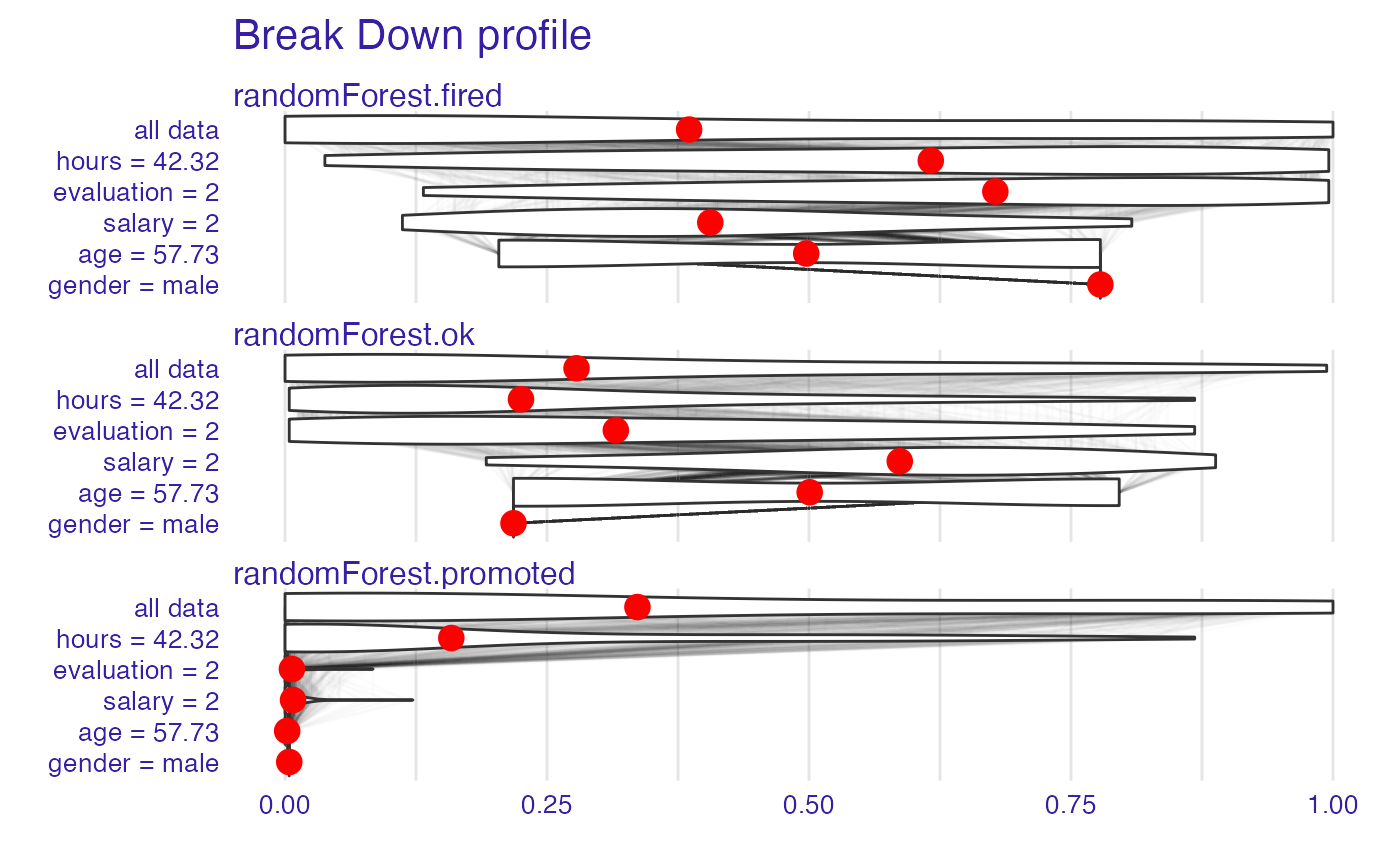bd_rf <- local_interactions(explainer_rf, new_observation, keep_distributions = TRUE) bd_rf #> contribution #> randomForest.fired: intercept 0.846 #> randomForest.fired: salary = 2 -0.352 #> randomForest.fired: age = 57.73 0.396 #> randomForest.fired: evaluation = 2 -0.084 #> randomForest.fired: hours = 42.32 -0.028 #> randomForest.fired: gender = male 0.000 #> randomForest.fired: prediction 0.778 #> randomForest.ok: intercept 0.990 #> randomForest.ok: salary = 2 -0.632 #> randomForest.ok: hours:age = 42.32:57.73 -0.154 #> randomForest.ok: gender = male 0.574 #> randomForest.ok: evaluation = 2 0.000 #> randomForest.ok: prediction 0.218 #> randomForest.promoted: intercept 0.990 #> randomForest.promoted: salary = 2 -0.588 #> randomForest.promoted: evaluation = 2 -0.304 #> randomForest.promoted: age = 57.73 0.802 #> randomForest.promoted: hours = 42.32 -0.122 #> randomForest.promoted: gender = male 0.000 #> randomForest.promoted: prediction 0.004plot(bd_rf)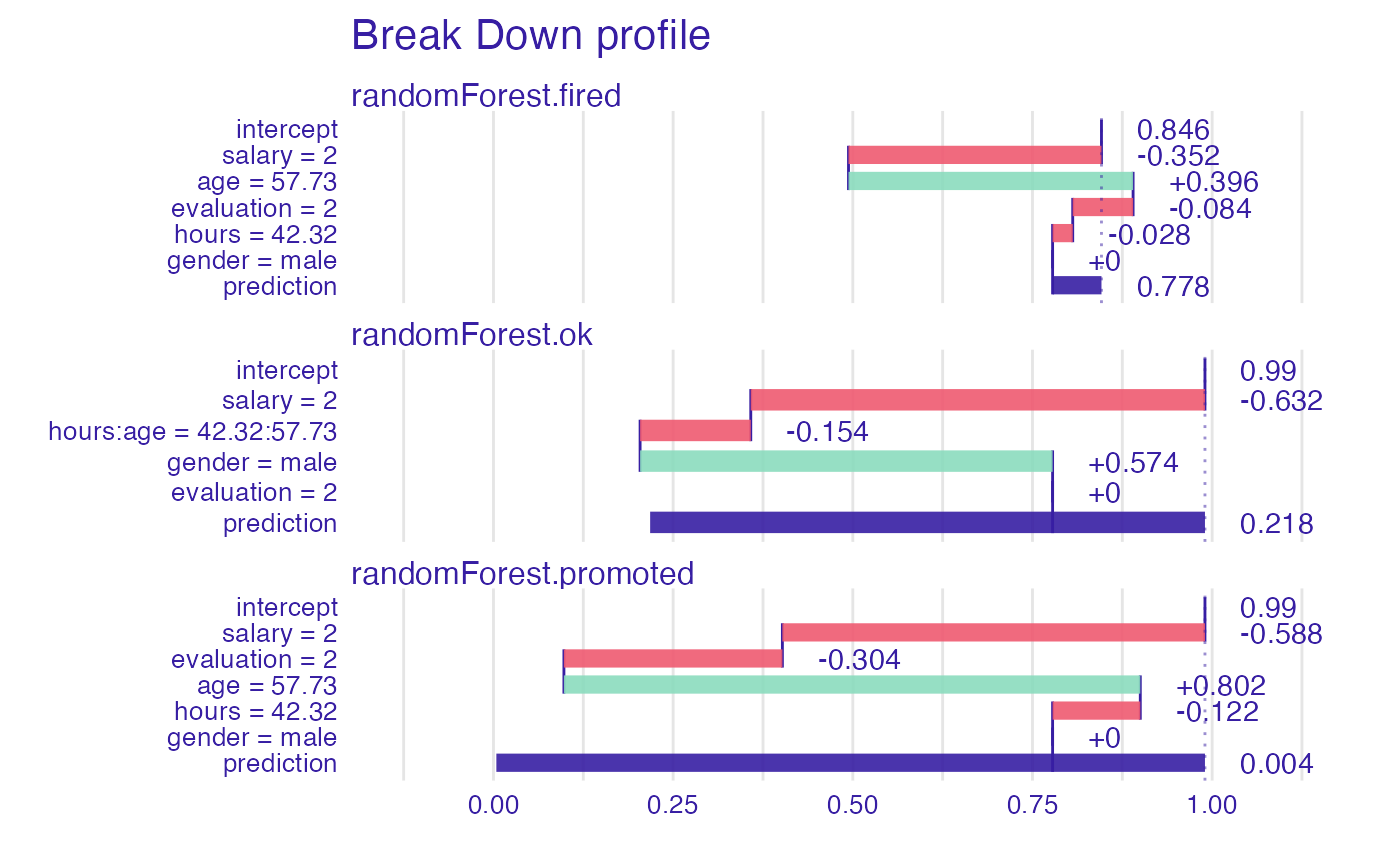plot(bd_rf, plot_distributions = TRUE) #> Warning: fun.y is deprecated. Use fun instead.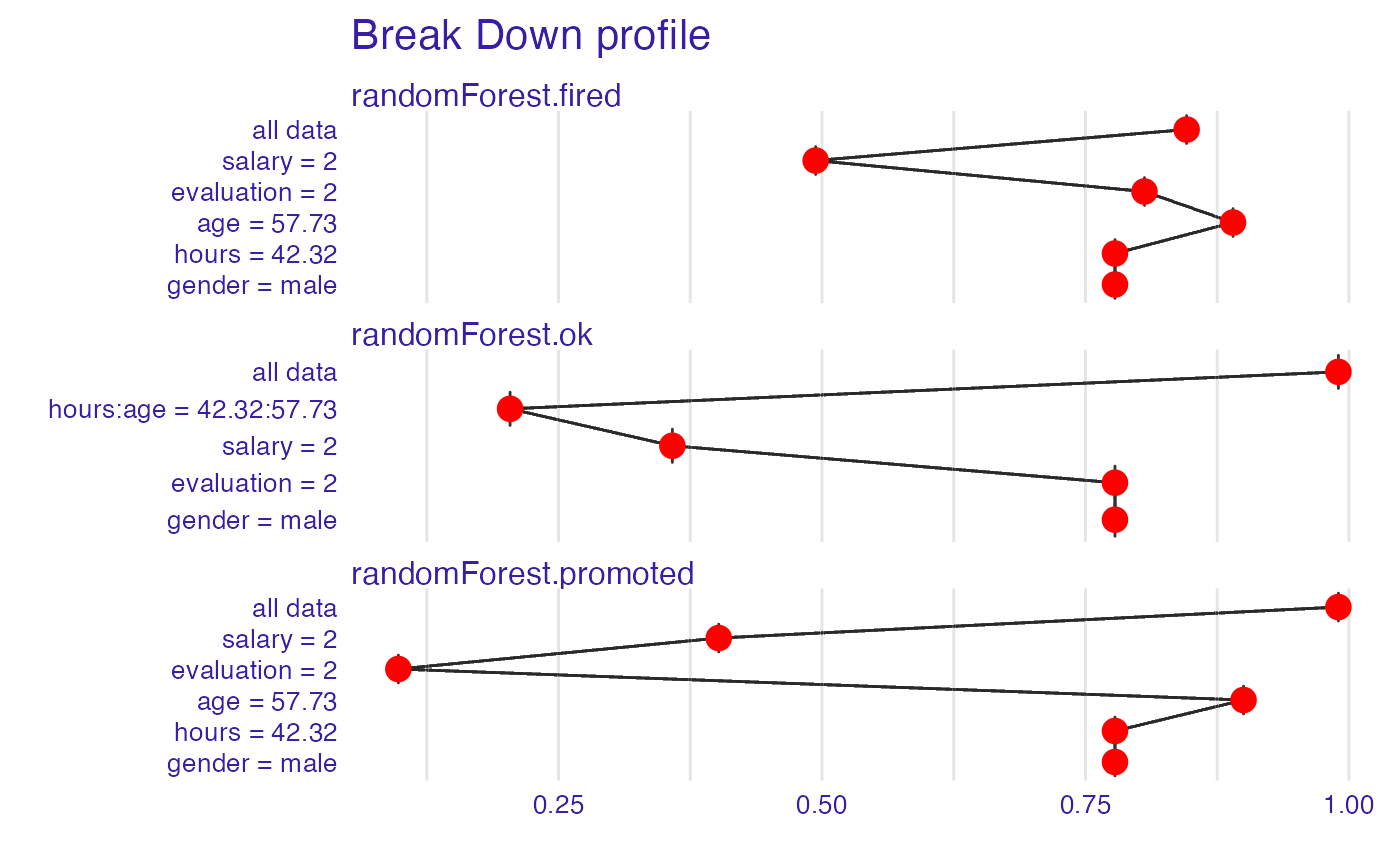# example for regression - apartment prices # here we do not have intreactions model <- randomForest(m2.price ~ . , data = apartments) explainer_rf <- explain(model, data = apartments_test[1:1000,2:6], y = apartments_test$m2.price[1:1000])
#> Preparation of a new explainer is initiated
#>   -> model label       :  randomForest  (  default  )
#>   -> data              :  1000  rows  5  cols
#>   -> target variable   :  1000  values
#>   -> predict function  :  yhat.randomForest  will be used (  default  )
#>   -> predicted values  :  No value for predict function target column. (  default  )
#>   -> model_info        :  package randomForest , ver. 4.6.14 , task regression (  default  )
#>   -> predicted values  :  numerical, min =  2043.066 , mean =  3487.722 , max =  5773.976
#>   -> residual function :  difference between y and yhat (  default  )
#>   -> residuals         :  numerical, min =  -630.6766 , mean =  1.057813 , max =  1256.239
#>   A new explainer has been created!
apartments_test[1,])
bd_rf
#>                                        contribution
#> randomForest: intercept                    3487.722
#> randomForest: district = Srodmiescie       1034.737
#> randomForest: surface = 131                -315.991
#> randomForest: no.rooms = 5                 -163.113
#> randomForest: floor = 3                     150.529
#> randomForest: construction.year = 1976      -24.021
#> randomForest: prediction                   4169.863plot(bd_rf, digits = 1)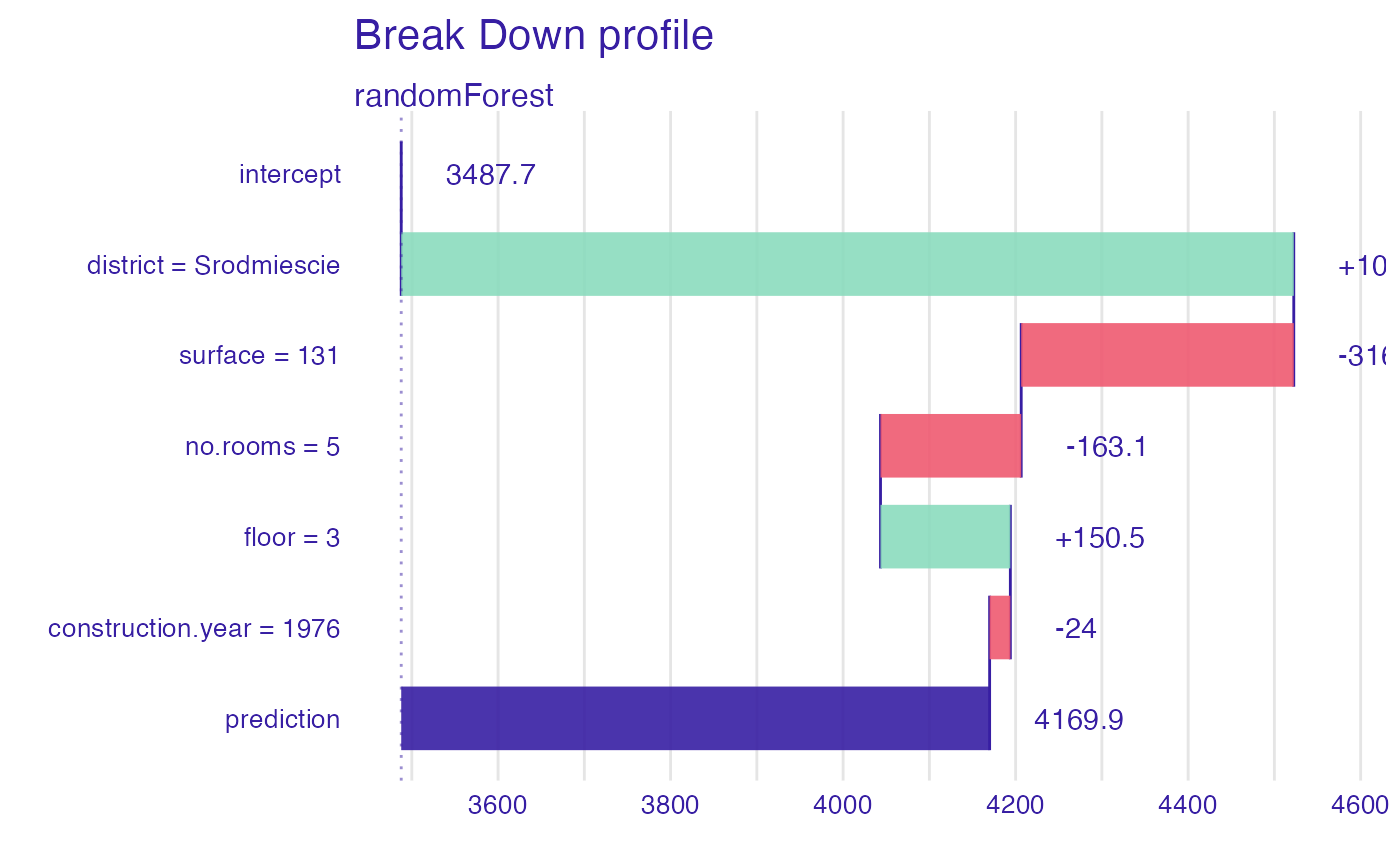plot(bd_rf, digits = 1, baseline = 0)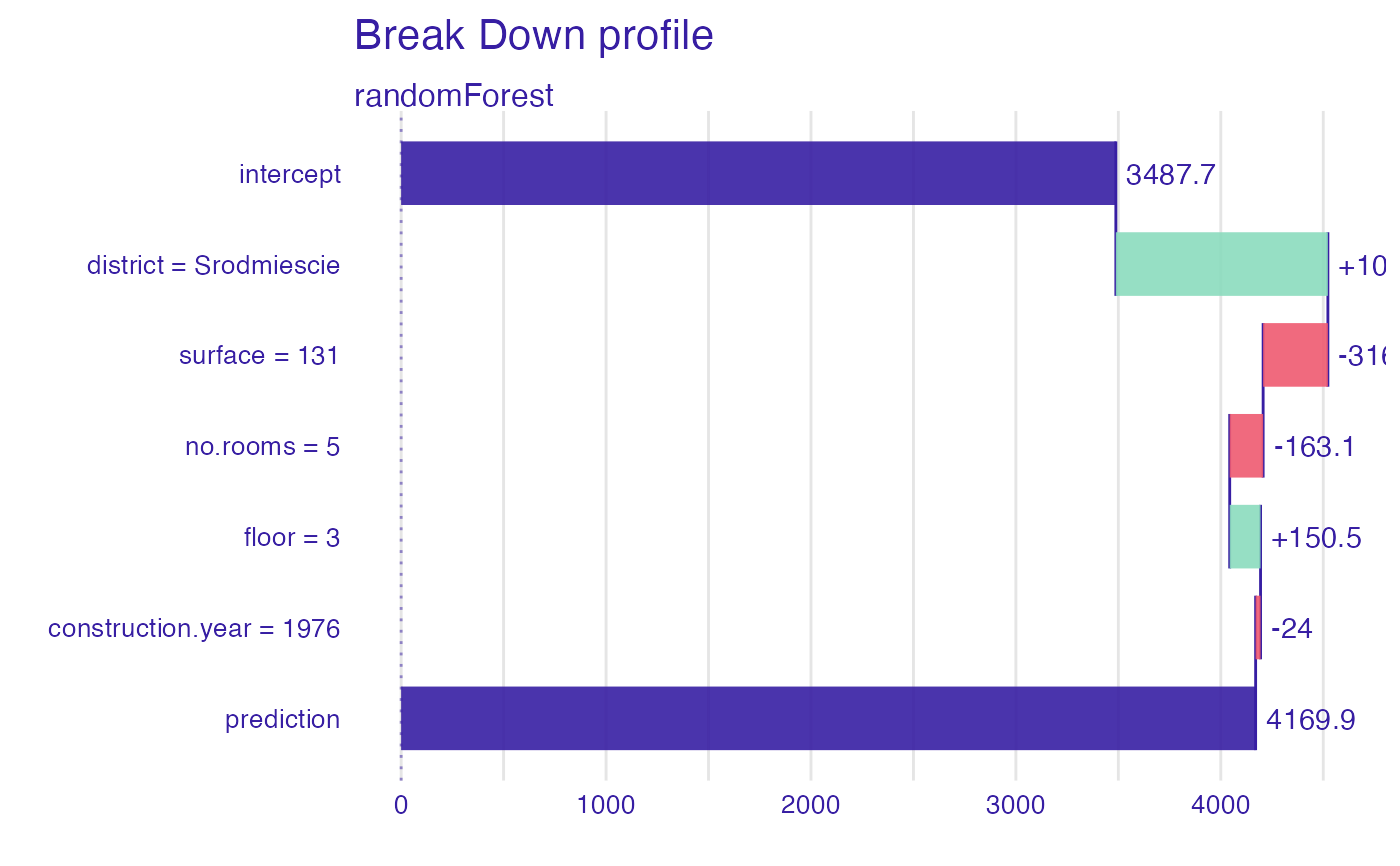apartments_test[1,],
keep_distributions = TRUE)
plot(bd_rf, plot_distributions = TRUE)
#> Warning: fun.y is deprecated. Use fun instead.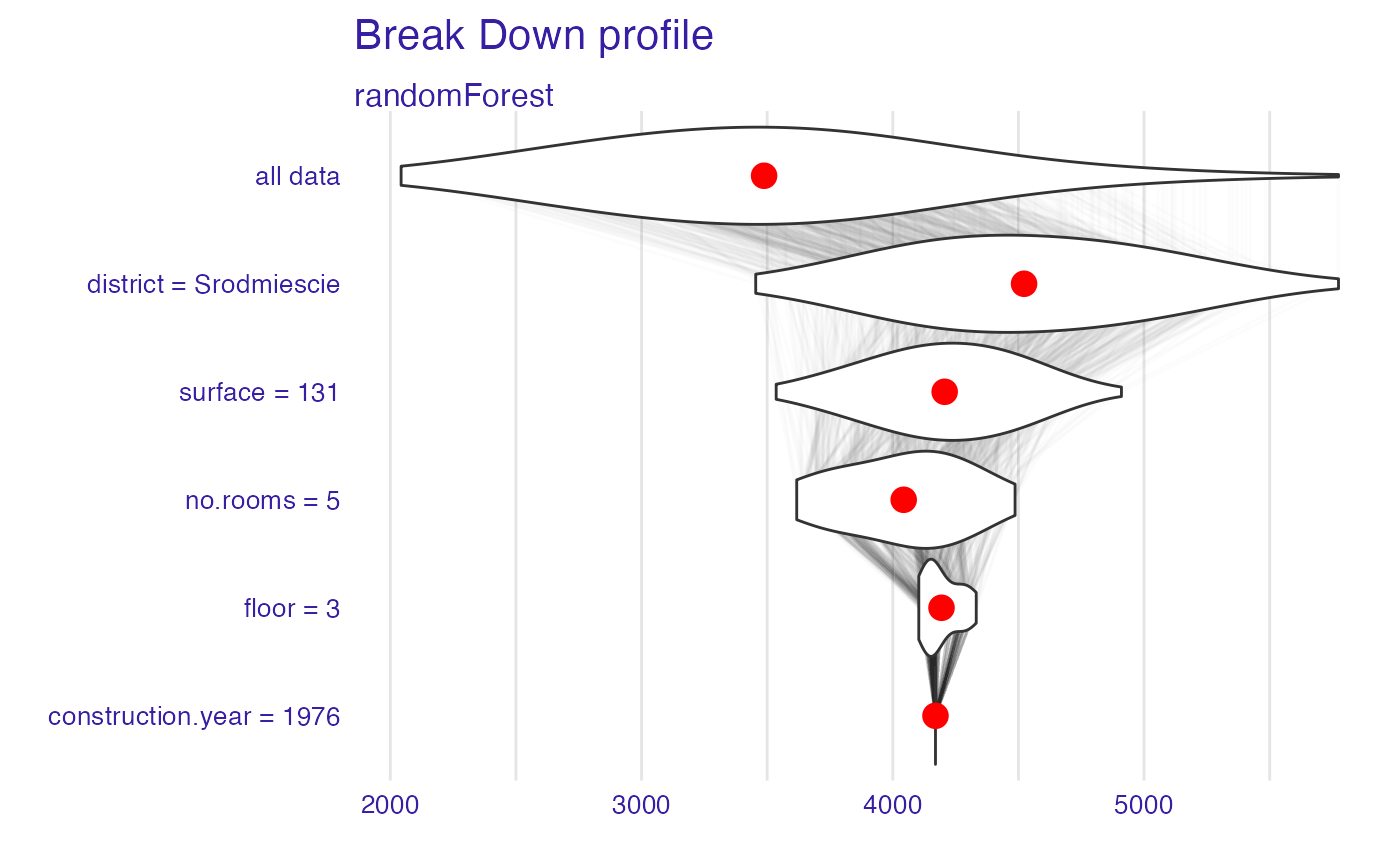bd_rf <- local_interactions(explainer_rf,
new_observation = apartments_test[1,],
keep_distributions = TRUE)

bd_rf
#>                                        contribution
#> randomForest: intercept                    3487.722
#> randomForest: district = Srodmiescie       1034.737
#> randomForest: surface = 131                -315.991
#> randomForest: no.rooms = 5                 -163.113
#> randomForest: floor = 3                     150.529
#> randomForest: construction.year = 1976      -24.021
#> randomForest: prediction                   4169.863plot(bd_rf)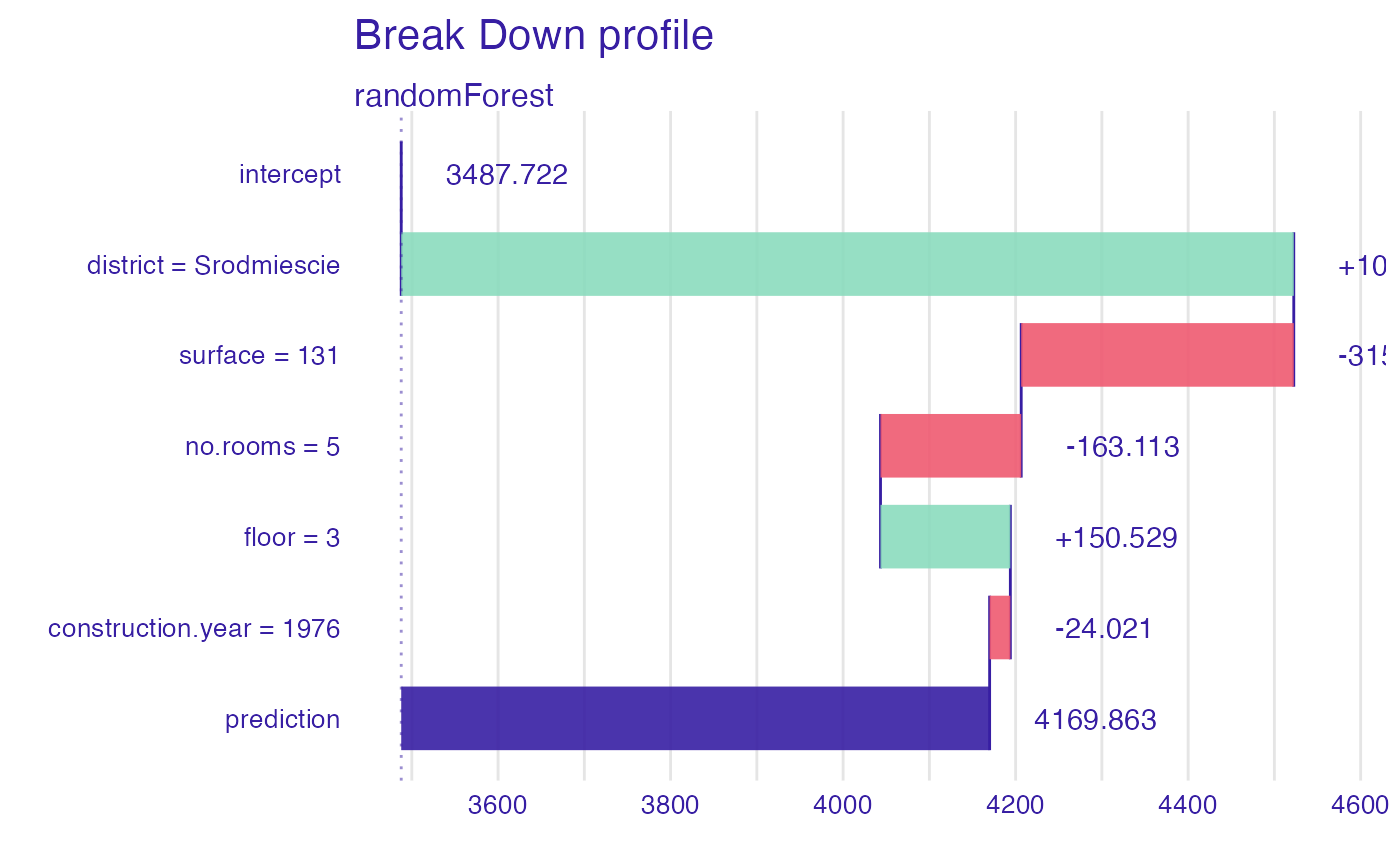plot(bd_rf, plot_distributions = TRUE)
#> Warning: fun.y is deprecated. Use fun instead.# }